Next: CLUSTEREASY: The Parallel Computing Up: Equations Previous: The Adiabatic Approximation

# The Staggered Leapfrog Method

LATTICEEASY uses a method called staggered leapfrog'' for solving differential equations. In order to solve a second order (in time) equation you need to store the value of the variable and its first time derivative at each step, and use these to calculate the value of the second time derivative. The idea of staggered leapfrog is to store the variables (i.e. the field values) and their derivatives at different times. Specifically, if the program is using a time stepand the field values are known at a time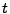then the derivatives will initially be known at a time. Using the field values the program can then calculate the second derivativeat timeand use this to advanceto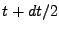. This value ofcan in turn be used to advance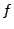to, thus restarting the process. Schematically, this looks like(6.95)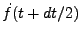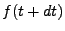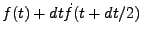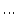Because each step advancesorin terms of its derivative at a time in the middle of the step this method has higher order accuracy and greater stability than a simple Euler method. However, the method relies on being able to calculatein terms ofat the time, so both accuracy and stability are generally lost ifdepends on the first derivative. This is the reason we choose our rescalings so as to eliminate first derivative terms in the equations of motion. Note that the evolution equation for the scale factor does have a first derivative in it. Section 6.2.2 describes how this problem is solved in the program.

To set the initial conditions for the staggered leapfrog calculations the field values and derivatives must be desynchronized. The initial conditions are set at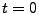and then the fields are advanced by an Euler step of size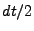to begin the leapfrog. Thereafter all calculations are done in full, staggered steps.

When the program saves output data, however, it is useful to synchronize the data again. The save() routine is always called when the field values are ahead of the field derivatives, so the function begins by moving the field values backwards by a half step, then calculating and saving all output quantities, and then moving the field values forward again. The one output function that does not use this technique is the checkpointing function that saves an image of the grid. This image is saved with the field values and derivatives desynchronized so that they can be read in again and used to continue the leapfrog calculation.Next: CLUSTEREASY: The Parallel Computing Up: Equations Previous: The Adiabatic Approximation